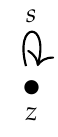Jonathan wrote:

> If we do this again, what do we get?

I'm not sure what "do this again" means. I would love to get [hyperoperations](https://en.wikipedia.org/wiki/Hyperoperation#Examples) this way. But there are some puzzling features.

Note that addition and multiplication are associative but exponentiation is not. Composition of morphisms is automatically associative. So, \$$(\mathbb{N},+,0)\$$ and \$$(\mathbb{N},\cdot,1)\$$ can be regarded as one-object categories but \$$(\mathbb{N},\uparrow,???)\$$ cannot, where \$$a \uparrow b = a^b\$$ is exponentation.

Exponentiation also lacks an identity, which is why I write \$$???\$$: we have \$$a\uparrow 1 = a\$$ but not \$$1 \uparrow a = a\$$.

For these reasons, we were able to get nice category-theoretic descriptions of
\$$(\mathbb{N},+,0)\$$ and \$$(\mathbb{N},\cdot,1)\$$, but the pattern must change a bit when we hit exponentation and higher hyperoperations:

* \$$(\mathbb{N},+,0)\$$ is isomorphic to \$$\mathbf{N}\$$, the free category on the graph* \$$(\mathbb{N},\cdot,1)\$$ is isomorphic to a category I'll call \$$\mathbf{M}\$$ with \$$\mathbf{N}\$$ as its only object and functors from \$$\mathbf{N}\$$ to itself as morphisms.

We could try to charge ahead naively:

**Puzzle.** What is the category with \$$\mathbf{M}\$$ as its only object and functors from \$$\mathbf{M}\$$ to itself as morphisms?

This could be interesting, and we could keep repeating this. But you are wisely taking a detour: to get exponentation, you're looking at functors from \$$\mathbf{N}\$$ to \$$\mathbf{M}\$$. What does it mean to "do this again"?